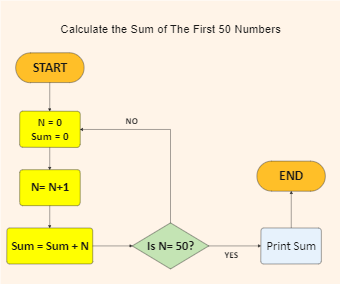Template Community / Calculate the Sum Algorithm Flowchart

# Calculate the Sum Algorithm FlowchartAshley
Published on 2021-09-27
Edit OnlineStep 1: Declare number N= 0 and sum= 0 Step 2: Determine N by N= N+1 Step 3: Calculate the sum by the formula: Sum= N + Sum. Step 4: Add a loop between steps 2 and 3 until N= 50. Step 5: Print Sum.
Tag
algorithm flowchart
Report
0
337PostRecommended Templates